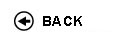Politecnico di Torino
Academic Year 2017/18
06BPTMC, 06BPTMQ
Analytical Mechanics
1st degree and Bachelor-level of the Bologna process in Civil Engineering - Torino
1st degree and Bachelor-level of the Bologna process in Mathematics For Engineering - Torino
 Teacher Status SSD Les Ex Lab Tut Years teaching Tosin AndreaA2 MAT/07 50 30 0 0 4
 SSD CFU Activities Area context MAT/07 8 A - Di base Matematica, informatica e statistica
 Subject fundamentals The main goal of the course is to introduce students to the mathematical theory of Mechanics as a prototype of the rigorous analytical formalisation of physical phenomena. The main topics treated in the course are the mechanics of the rigid body and of the articulated systems and the Lagrangian mechanics. Expected learning outcomes Students will learn to deal with mathematical models of mechanical systems and with the related mathematical methods of qualitative analysis. Prerequisites / Assumed knowledge Concepts and methodologies from the courses of Calculus I, II and Geometry. Contents - Revision of vector calculus in R^3. Inner product and modulus, bases and components, change of basis, vector product, triple product, applied vectors, centre of parallel vectors. - Revision of differential geometry: curves and surfaces. Arc length, intrinsic reference system (tangent, normal and binormal unit vectors), parametrisation of a surface, tangent space. - Kinematics of a point particle. Equation of motion and trajectory, velocity and acceleration. - Kinematics of the rigid body. Rigidity constraint, rigid configurations, Poisson theorem and angular velocity, velocity and acceleration fields of a rigid motion, particular rigid motions (translational, roto-translational, rotational, planar), instant centre of rotation for planar motions, Chasles theorem. - Relative kinematics. Derivative of a vector with respect to two observers, Galileo theorem, Coriolis theorem, composition of angular velocities. - Constrained systems. Classification of the constraints (holonomic, nonholonomic, bilateral, unilateral, time-independent, time-dependent), Lagrangian coordinates and degrees of freedom, virtual velocities and displacements. - Geometry of areas and masses. Geometrical characteristics of planar sections, barycentre, centre of mass, statical moments of areas, moments of inertia, Huygens-Steiner theorem, matrix of inertia, principal axes of inertia. Examples of geometrical characteristics of special planar sections (rectangle, square, circle, sections composed by rectangles: T-section, double T-section, U-section). - Fundamental equations of Dynamics. Systems of forces, resultant and torque, work and virtual work of a force, work of forces on a holonomic system, generalised forces, work of forces on a rigid system, dynamics of a point particle, reactive forces, ideal constraints, linear momentum, angular momentum, first fundamental equation of Dynamics, second fundamental equation of Dynamics, angular momentum and its time derivative for rigid systems, Euler equations, first integrals. - Statics and equilibria. First fundamental equation of Statics, second fundamental equation of Statics, principle of virtual works, statics of rigid systems, statics of holonomic systems, equilibrium configurations, potential, principle of stationary potential, stability. - Kinetic energy. Koening theorem, kinetic energy for a rigid and a holonomic system, mass matrix, power of forces, theorem of the kinetic energy, principle of conservation of mechanical energy. - Lagrangian mechanics. Lagrange equations for holonomic systems, Lagrangian, first integral of canonical momenta. - Small oscillations. Linearisation of Lagrange equations, characteristic frequencies of oscillation. Delivery modes The course is organised in: - theoretical lectures (50 hours); - classroom exercises (30 hours). During classroom exercises students are exposed to the guided solution of problems focussed on the following topics: - kinematics of free and constrained point particles and rigid bodies; - relative kinematics; - calculation of the relevant geometrical characteristics of planar sections; - fundamental equations of dynamics; - fundamental equations of statics; - principle of virtual works, principle of stationary potential; - calculation of the equilibrium configurations of a mechanical system and discussion of their stability; - calculation of dynamical and statical reactive forces; - Lagrange equations; - linearisation of the Lagrange equations about stable equilibrium configurations. During the last part of the course, classroom exercices will also focus on the solution of exercises from past exams. Texts, readings, handouts and other learning resources P. Biscari, T. Ruggeri, G. Saccomandi, M. Vianello, Meccanica Razionale (3a edizione), Springer, 2016 Assessment and grading criteria The examination consists in a 120-minute written exam, which assesses the theoretical knowledge of the students and their ability to deal qualitatively with mechanical systems. The examination is composed of two parts: - a theoretical open-ended question (5 points); - an exercise on the topics taught during the lectures and the pratical classes (26 points). The maximum score obtainable from the written exam is 31, corresponding to the grade 30 cum laude. During the written exam students may consult a form that they can fill in personally in advance not exceeding one side of an A4-size sheet. An oral exam, integrating the written one, is possible upon request of the students (if satisfactory in the written part) or of the teacher. The oral exam consists in theoretical questions, which may include both the proof of theorems and the application of solution techniques developed during the course. If requested, the oral exam contributes to the final grade of the examination together with the written exam. In particular, it can either increase or decrease the score obtained from the written exam within the full range [0, 30] of available grades. Programma definitivo per l'A.A.2017/18© Politecnico di Torino
Corso Duca degli Abruzzi, 24 - 10129 Torino, ITALY